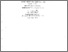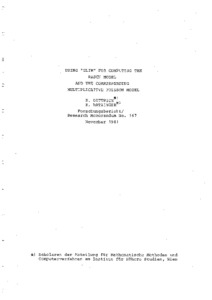# using 'glim' for computing the rasch model and the corresponding multiplicative poisson model

Dittrich, Regina and Hatzinger, Reinhold (November 1981) using 'glim' for computing the rasch model and the corresponding multiplicative poisson model. Closed Series > Forschungsberichte / Research Memoranda 167Preview
Text
fo167.pdf

Download (975kB) | Preview

## Abstract or Table of Contents

abstract: randomising the row marginals of rasch's dichotomous logistic model (rm) leads to an extended randomised version of the rm under assumption of free variability of the marginal probabilities on the surface of a corresponding probability simplex. the resulting model is shown to be widely identical to a multiplicative poisson model. the part of the ml-equation for estimating the item parameters coincides with the conditional maximum likelihood of the rm. the connection of these models isformulated in the context of generalised linear models which provides the possibility to use the glim-package for computing numerical solutions for a moderate number of items. the general structure of the models is discussed by an example. additionally the paper contains the presentation of glim-macros and directives required for individual applications.;

Item Type: IHS Series Published 26 Sep 2014 10:34 01 Apr 2016 14:07 https://irihs.ihs.ac.at/id/eprint/167

### Actions (login required)View Item